## Code and Output

### Code 11.1.1:

``````figure(1)
clf
for loopvalue = 2:2:38
thisx = 1 + loopvalue/10;
thisy = 2 - loopvalue/20;
thissize = loopvalue;
plot(thisx,thisy,'*','Markersize', thissize);
hold off
axis([1 5 0 2])
pause(.1);
end``````

### Code 11.1.2:

``````close all
clear all
shg

ShoulderX = 0;
ShoulderY = 0;

moves = 6;
want_animation = true;

ShoulderAngle = linspace(.15*pi,.45*pi,moves);
ElbowAngle = linspace(.75*pi,.55*pi,moves);

position = [];

figure(1)
hold on; grid on; box on;
xlim([-2.5 2.5]);
ylim([-2.5 2.5]);

for i = 1:moves
ElbowX(i) = ShoulderX + cos(ShoulderAngle(i));
ElbowY(i) = ShoulderY + sin(ShoulderAngle(i));

HandX(i) = ElbowX(i) + cos(ElbowAngle(i));
HandY(i) = ElbowY(i) + sin(ElbowAngle(i));

position = [position; [ShoulderX ElbowX(i) HandX(i)] ...
[ShoulderY ElbowY(i) HandY(i)]];
armhandle = plot(position(i,1:3),position(i,4:6),'ko-');
if want_animation
pause(.2)
if i < moves
delete(armhandle);
end
end
end
if not(want_animation)
saveas(gcf,'Output_11_1_2','eps')
end``````

### Output 11.1.2: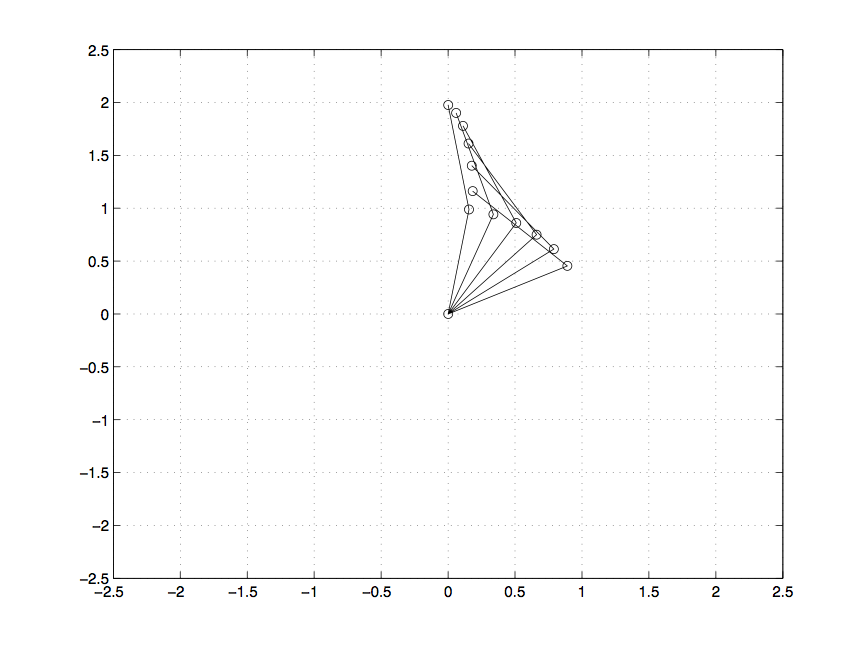### Code 11.3.1:

``````figure(2)
shg
hold off
for i = 1:moves
plot(position(i,1:3),position(i,4:6),'ko-');
grid on
xlim([-2.5 2.5]);
ylim([-2.5 2.5]);
drawnow
pause(.3)
end
close(2)``````

### Code 11.4.1:

``````grid on
box on
moves = 6;
for i = 1:moves
plot(position(i,1:3),position(i,4:6),...
'ko-','erasemode','normal');
xlim([-2.5 2.5]);
ylim([-2.5 2.5]);
F(i) = getframe;
end
pause(1)
movie(F,1)``````

### Code 11.5.1:

``movie2avi(F,'ArmMove.avi')``

### Code 11.5.2:

``````% Write the movie backwards
figure(3);
clf
writerObj = VideoWriter('evoMmrA'); % Release 2013a or later
open(writerObj)
for k = 6:-1:1
image(F(k).cdata)
frame = getframe;
writeVideo(writerObj,frame);
end
close(writerObj)  % 'evommrA.avi' is readable in Mac OS, too``````

### Code 11.6.1:

``````myMovieObj = VideoReader('shuttle.avi');
nFrames = myMovieObj.NumberOfFrames;
for k = 1 : nFrames
end
for k = 1:10:nFrames
image(myFrames(k));shg;
text(50,50,sprintf('Frame Number: %d',k));
pause(0.5);
end``````

### Code 11.6.2:

``````xyloObj = VideoReader('shuttle.avi');
nFrames = size(vidFrames,4);
figure(4)
writerObj = VideoWriter('elttuhs.avi');
open(writerObj);
for k = nFrames:-1:1
image(vidFrames(:,:,:,k))
frame = getframe;
writeVideo(writerObj,frame);
end
close(writerObj)``````

### Code 11.6.3:

``````im = vidFrames(:,:,:,6);
figure(2)
image(im)
axis image``````

### Code 11.7.1:

``````beep
pause(2)
beep;``````

### Code 11.8.1:

``````load chirp
sound(y)
plot(y,'k')
commandwindow
whos
Fs``````

### Output 11.8.1: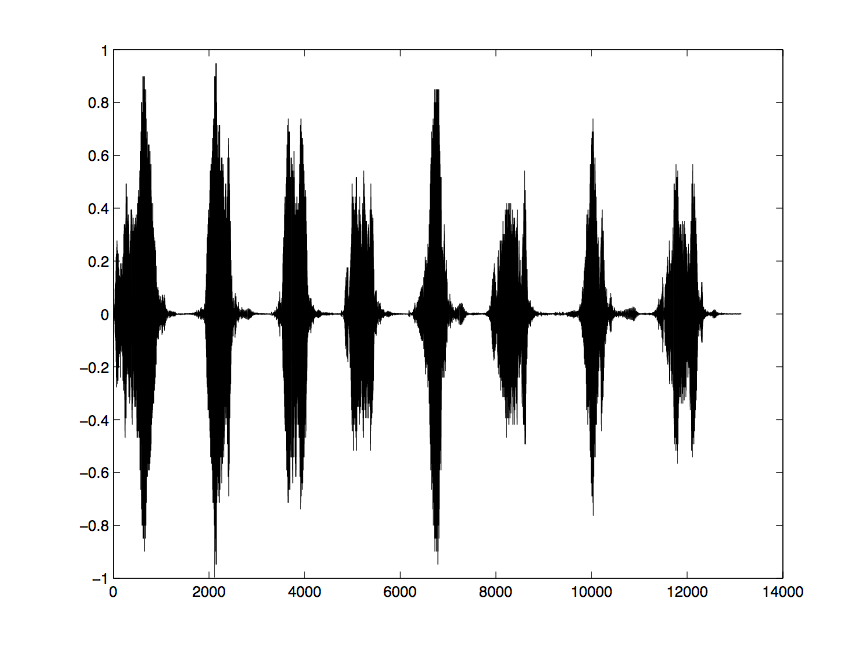### Output 11.8.2:

``````  Name          Size             Bytes  Class     Attributes

Fs            1x1                  8  double
y         13129x1             105032  double

Fs =
8192``````

### Code 11.9.1:

``````load handel
soundsc(y,[-3.25 3.25])
pause(9)
soundsc(y,[-15.25 15.25])``````

### Code 11.10.1:

``````load handel;
handelplayer = audioplayer(y,Fs);

chirpplayer = audioplayer(y,Fs);

play(handelplayer)
pause(2)
play(chirpplayer)``````

### Code 11.10.2:

``````playblocking(handelplayer)
pause(2)
playblocking(chirpplayer)``````

### Code 11.10.3:

``````t = timer('TimerFcn','play(handelplayer)', ...
'StartDelay', 1.5);
start(t)``````

### Code 11.11.1:

``````load gong;   % Loads y and Fs for sound from gong.mat
tooloudplayer = audioplayer(y,Fs);  % Volume is too loud!
toosoftplayer = audioplayer(y/5,Fs);  % Volume is too soft!
goldilocksplayer = audioplayer(y/2,Fs); %Volume is just right!

playblocking(tooloudplayer);
playblocking(toosoftplayer);
playblocking(goldilocksplayer);``````

### Code 11.12.1:

``````sf = 22050;                      % sample frequency
d = 1.0;                         % duration
n = sf*d;                        % number of samples
noise = rand(1,n);               % uniform distribution
noise = noise / max(abs(noise)); % normalize
sound(noise,sf);                 % play sound
subplot(2,1,1)
plot(noise,'k')
xlim([1 n]);
subplot(2,1,2)
plot(noise(1:250),'k')``````

### Output 11.12.1: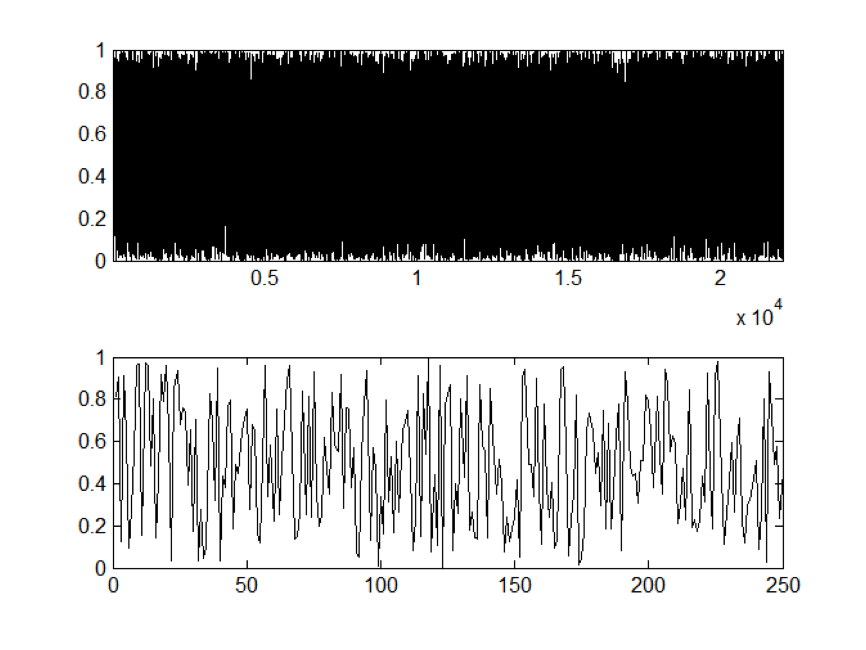### Code 11.12.2:

``````cf = 440;                  % carrier frequency (Hz)
sf = 22050;                      % sample frequency (Hz)
d = 1.0;                         % duration (s)
n = sf * d;                      % number of samples
s = (1:n) / sf;                  % time-dependent values
tone = sin(2 * pi * cf * s);     % sinusoidal modulation
sound(tone,sf);                  % sound presentation
subplot(2,1,1)
plot(tone,'k')
subplot(2,1,2)
plot(tone(1:250),'k')``````

### Output 11.12.2: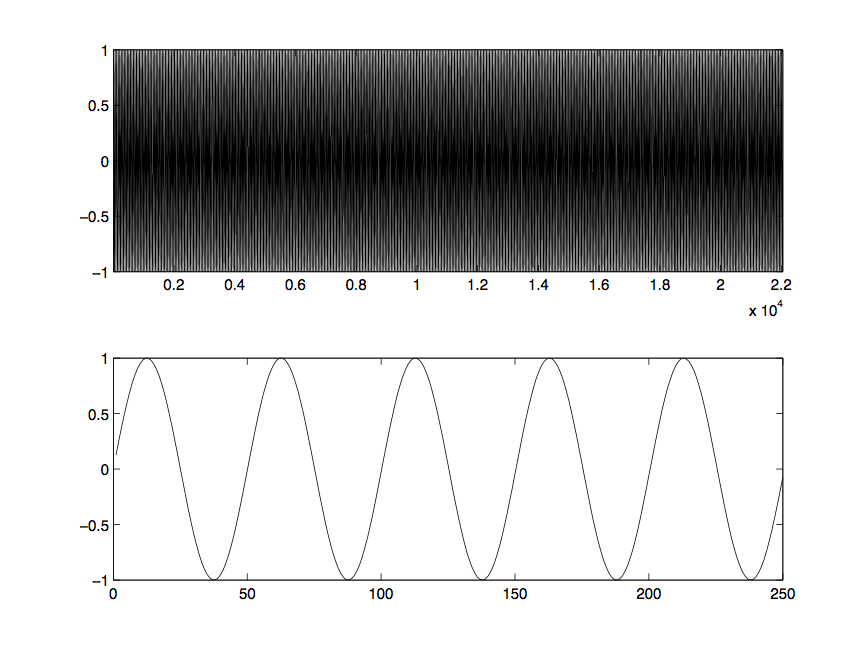### Code 11.12.3:

``````a = linspace(1/length(tone),1,length(tone));
sound(a.*tone,sf)
plot(a.*tone,'k')
subplot(2,1,1)
plot(a.*tone,'k')
xlim([1 n]);
subplot(2,1,2)
plot(a(1:5000).*tone(1:5000),'k')
ylim([-1 1]);``````

### Output 11.12.3: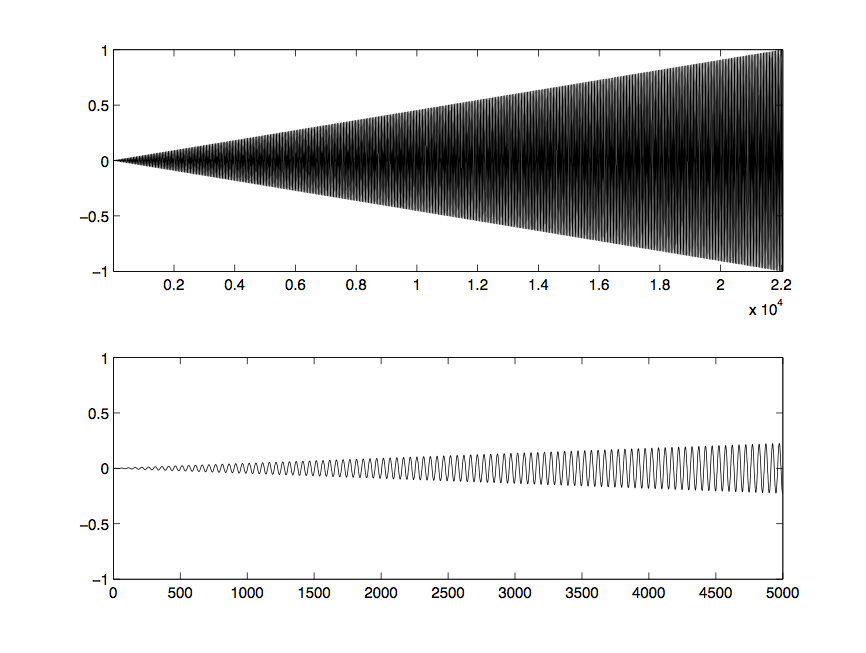### Code 11.12.4:

``````    fs = 8000;       % sampling frequency
t = 0:1/fs:0.25; % length of each note
tspace = 1.0;    % length of pause between notes
fr = 2^(1/12);   % frequency ratio between neighboring keys
A4 = 440;        % reference note for others
B4 = A4*fr^2;
C4 = A4*fr^(-9);
D4 = A4*fr^(-7);
E4 = A4*fr^(-5);
F4 = A4*fr^(-4);
G4 = A4*fr^(-2);
C5 = A4*fr^3;
xspace = zeros(1,tspace*fs);    % set pause
x = [cos(C4*2*pi*t),xspace, ...
cos(D4*2*pi*t),xspace, ...
cos(E4*2*pi*t),xspace, ...
cos(F4*2*pi*t),xspace, ...
cos(G4*2*pi*t),xspace, ...
cos(A4*2*pi*t),xspace, ...
cos(B4*2*pi*t),xspace, ...
cos(C5*2*pi*t)];
myScale = audioplayer(x,fs);
play(myScale)
audiowrite('scale.wav',x,fs)``````

### Code 11.13.1:

``````[y,Fs] = audioread('scale.wav');
sound(y,Fs)``````

## Solutions

``````% Solutions_Chapter_11

% Solutions for selected problems from MATLAB for Behavioral Scientists,
% Second Edition (D. A. Rosenbaum, J. Vaughan, & B. Wyble),
% (c) 2015, Taylor & Francis

% To generate the solution for one problem, copy and run the code for that
% problem in a file or paste it into the Command window. Show the Command
% window to see the results.

% To generate sll the solutions for Chapter 11, save this code as a
% MATLAB script file and run the program.

% Solutions with animated output (11.15.1 and 11.15.2), and with audio
% output (11.15.6 amd 11.15.8) must be run in a MATLAB script file. The
% animation and sound will not be apparent in the web version.

function main % Problems in this chapter may have nested or local
% functions so the Problem 11 file must itself be a function
close all
clc
commandwindow
Solution_11_15_1 %Run each of the problem functions in turn.
Solution_11_15_2
Solution_11_15_3
Solution_11_15_4
Solution_11_15_5
Solution_11_15_6
Solution_11_15_7
Solution_11_15_8
Solution_11_15_9
Solution_11_15_10
Solution_11_15_11
end % function main

% Problem 11.15.1

% Write an animation program to show the view from the flight deck of a
% starship entering Warp Speed, so that the figure shows an expanding
% optic flow field of multiple objects, looming closer. Each point will
% appear to grow in size as it follows a straight trajectory toward the
% edge of the screen. (Hint: After you have plotted an array of points
% use GET in a loop to change the size and position of an array
% of points).

function Solution_11_15_1
close all;
fprintf('\n\n          %s\n\n','Output 11.15.1  [Animated]')
figure('name','Output.11.15.1')
nstars = 1:10;
for npoints = nstars
orientation = rand * 2*pi;
distance = 20 + randi(70);
x = distance * sin(orientation);
y = distance * cos(orientation);
thismarkersize = max(abs([x y]))/10;
starhandle(npoints) = plot(x,y,'r*','Markersize',...
thismarkersize);hold on;
end
shg;
for cycles = 1:100;
for npoints = nstars
x = get(starhandle(npoints),'XData');
y = get(starhandle(npoints),'YData');
x = x * 1.1;
y = y * 1.1;
% [x y]
if (abs(x) < 100) && (abs(y) < 100)
%Not to boundary yet so move the point and make it grow
set(starhandle(npoints),'XData',x);
set(starhandle(npoints),'YData',y);
thismarkersize = max(abs([x y]))/10;
set(starhandle(npoints),'Markersize',thismarkersize);
else
%     disp([x y])
% At boundary, so make a new star using the same handle
orientation = rand * 2*pi;
distance = 20;
x = distance * sin(orientation);
y = distance * cos(orientation);
%     [x y]
thismarkersize = max(abs([x y]))/10;
set(starhandle(npoints),'XData',x);
set(starhandle(npoints),'YData',y);
set(starhandle(npoints),'Markersize',thismarkersize);
end
axis([-100 100 -100 100]);

end
drawnow;
title('Output 11.15.1');
end
end

% Problem 11.15.2
%
% Adapt the program used to generate the motion of a right arm
% (Code 11.1.2 and 11.3.1) so the left arm and right arm both move
% at once.
% Save the output as a movie so it can be viewed outside MATLAB.

function Solution_11_15_2
fprintf('\n\n          %s\n\n','Output 11.15.2 [Animated]')
figure(2)
set(2,'name','Output.11.15.2')
clear all
ShoulderX = 0;
ShoulderY = 0;

moves = 6;
want_animation = true;

ShoulderAngle = linspace(.15*pi,.45*pi,moves);
ElbowAngle = linspace(.75*pi,.55*pi,moves);

position = [];

hold on; grid on; box on;
xlim([-2.5 2.5]);
ylim([-2.5 2.5]);

for i = 1:moves
ElbowX(i) = ShoulderX + cos(ShoulderAngle(i));
ElbowY(i) = ShoulderY + sin(ShoulderAngle(i));

HandX(i) = ElbowX(i) + cos(ElbowAngle(i));
HandY(i) = ElbowY(i) + sin(ElbowAngle(i));

position = [position; [ShoulderX ElbowX(i) HandX(i)] ...
[ShoulderY ElbowY(i) HandY(i)]];
armhandle = plot(position(i,1:3),position(i,4:6),'ko-');
if want_animation
pause(.2)
if i < moves
delete(armhandle);
end
end
end
title('Output 11.15.2');
if not(want_animation)
saveas(gcf,'Output_11_15_2','eps')
end

figure(3)
hold off
% Make a left arm mirror image of the right arm
position = position + .5;
lposition = - position;

% lposition(:,1:3) =   - .5    -    (lposition(:,1:3) - .5);
for i = 1:moves
clf;
plot(position(i,1:3),position(i,4:6),'ro-');hold on
plot(lposition(i,1:3),position(i,4:6),'ko-');
grid on
xlim([-1.5 1.5]);
ylim([0 3]);
mymovie(i) = getframe;
hold off;
end
movie(mymovie,1)

% Create movie myarms.avi using movie2avi
movie2avi(mymovie, 'myarms')
% myarms.avi requires VLC to play on a Mac

% Create movie myarms.avi using VideoWriter
writerObj = VideoWriter('myarms2');
open(writerObj);
for i = 1:moves
plot(position(i,1:3),position(i,4:6),'ro-');hold on
plot(lposition(i,1:3),position(i,4:6),'ko-');
grid on
xlim([-1.5 1.5]);
ylim([0 3]);
thismovieframe = getframe;
hold off;
writeVideo(writerObj,thismovieframe);
end
close(writerObj);
title('Output 11.15.3');
% The file you created, "myarms2.avi" can now be played on a PC
% myarms2.avi requires QuickTime to play on a Mac

end

%
% Problem 11.15.3
%
% % Adapt the program used to generate the motion of a right arm so one
% % arm or both arms (as you wish) reach out to contact a moving ball.
% % Save the output so it can be viewed outside MATLAB.

function Solution_11_15_3
fprintf('\n\n          %s\n','Output 11.15.3')
fprintf('Solution left to the student.\n\n');
end % function Solution_11_15_3

% Problem 11.15.4
%
% Write a program for an experiment on intermodal perception. For
% example, show an animation along with a sound sequence that either
% fits or does not fit with the animation. Such stimuli have been
% presented to infants to determine whether infant gaze durations
% depend on the match between visual and auditory stimuli, or to
% adults to determine the interactions of vision and audition in
% the attribution of causality when objects appear to collide.

function Solution_11_15_4
fprintf('\n\n          %s\n','Output 11.15.4')
fprintf('Solution left to the student.\n\n');
end

% Problem 11.15.5
%
% Write a program to read and run a previously saved movie either with
% the frames in their original order, in reverse order, or in some
% scrambled order. Save the output so it can be viewed outside MATLAB.

function Solution_11_15_5
fprintf('\n\n          %s\n','Output 11.15.5')
fprintf('Solution left to the student.\n\n');end % function Solution_11_15_5

% Problem 11.15.6
%
% Adapt Code 11.12.4 to play a melody such as "Twinkle, twinkle, little
% star."  Use functions so that you can specify the sounds economically
% (i.e as a string of note values, such as 'CCGGAAG'), and easily
% change the tune.

function Solution_11_15_6
fprintf('\n\n          %s\n\n','Output 11.15.6 [Audio]')
fs = 8000;       % sampling frequency
t = 0:1/fs:0.25; % length of each note
%     tspace = 1.0;    % length of pause between notes
fr = 2^(1/12);   % frequency ratio between neighboring keys

A4frequency = 440;
% reference frequency for notes
C4 = A4frequency*fr^(-9);
D4 = A4frequency*fr^(-7);
E4 = A4frequency*fr^(-5);
F4 = A4frequency*fr^(-4);
G4 = A4frequency*fr^(-2);
A4 = A4frequency;
B4 = A4frequency*fr^2;
C5 = A4frequency*fr^3;

notemap = [
'A' 'B' 'C' 'D' 'E' 'F' 'G'
A4 B4 C4 D4 E4 F4 G4];

tune = 'CCGGAAG'; % Twinkle Twinkle
for note = 1:length(tune)
% find frequency of note
thisnote = find((notemap(1,:) == tune(note)));
% construct note and play it
x = cos(notemap(2,thisnote)*2*pi*t);
mynote = audioplayer(x,fs);
playblocking(mynote);
end

end
%
% Problem 11.15.7
%
% Write a program to take a series of tones of different notes and
% durations and play it repeatedly, at a slow tempo, so you can play
% along as you are learning your musical instrument. Adjust the tempo
% as you master the lick.
%

function Solution_11_15_7
fprintf('\n\n          %s\n','Output 11.15.7')
fprintf('Solution left to the student.\n\n');
end

% Problem 11.15.8
%
% You may have noticed that there is a bit of a "click" between the
% tones, due to the abrupt transition from zero to full amplitude of
% the waveform. Devise a way for each sound to gradually begin and end,
% so the onset and offset of each sound are more gradual.
%
function Solution_11_15_8
fprintf('\n\n          %s\n','Output 11.15.8 [Audio]')
pause(2)
fs = 8000;       % sampling frequency
t = 0:1/fs:0.25; % length of each note
fr = 2^(1/12);   % frequency ratio between neighboring keys

A4frequency = 440;
% reference frequency for notes
C4 = A4frequency*fr^(-9);
D4 = A4frequency*fr^(-7);
E4 = A4frequency*fr^(-5);
F4 = A4frequency*fr^(-4);
G4 = A4frequency*fr^(-2);
A4 = A4frequency;
B4 = A4frequency*fr^2;
C5 = A4frequency*fr^3;
rest = 0;  %rest is a tone of frequency zero

notemap = [
'A' 'B' 'C' 'D' 'E' 'F' 'G' 'r'
A4 B4 C4 D4 E4 F4 G4 rest];

tune = 'EDCDEEErDDDrEGGrEDCDEEEEDDEDC'; % Mary had a little lamb
for note = 1:length(tune)
% find frequency of note
thisnote = find((notemap(1,:) == tune(note)));
x = cos(notemap(2,thisnote)*2*pi*t);
% Smooth onset and offset transients
% of notes by ramping volume up and down
myvolume = ones(1,length(x));
myvolume(1:300) = linspace(0,1,300);
myvolume(end:-1:end-299) = linspace(0,1,300);
%leave a zero-volume gap at the end of each note to eliminate click
mynote = audioplayer([x.*myvolume zeros(1,100)],fs);
playblocking(mynote);
end
end

% Problem 11.15.9
%
% Write a program for an experiment in which participants make auditory
% discriminations. For example, participants perform a forced choice
% task in which they indicate which of two tones is louder: the first
% or the second.

function Solution_11_15_9
fprintf('\n\n          %s\n','Output 11.15.9')
fprintf('Solution left to the student.\n\n');
end

% Problem 11.15.10
%
% Write a program in which subjects answer questions and get auditory
% feedback that indicates whether they got the answer right or wrong.
% To make things a bit fancy, change the volume of the sound according
% to how quickly the question was answered and according to whether the
% answer was right or wrong.

function Solution_11_15_10
fprintf('\n\n          %s\n','Output 11.15.10')
fprintf('Solution left to the student.\n\n');
end

% Problem 11.15.11
%
% Write a program for an experiment on intermodal perception. For
% example, show an animation along with a sound sequence that either
% fits or does not fit with the animation. Such stimuli have been
% presented to infants to determine whether infant gaze durations
% depend on the match between visual and auditory stimuli, or to
% adults to determine the interactions of vision and audition in
% the attribution of causality when objects appear to collide.

function Solution_11_15_11
fprintf('\n\n          %s\n','Output 11.15.11')
fprintf('Solution left to the student.\n\n');
end``````

### Output 11.15.1 [Animated]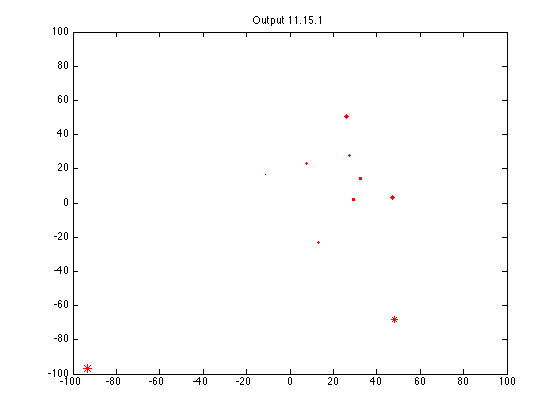### Output 11.15.2 [Animated]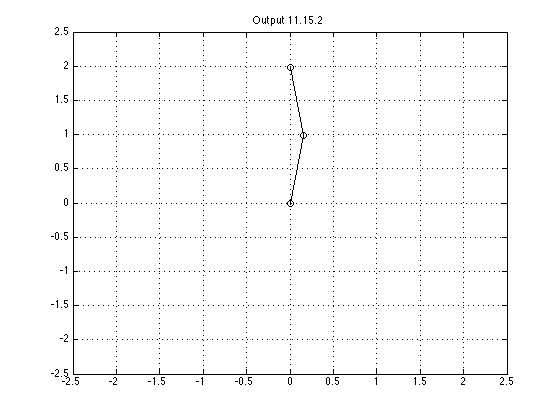### Output 11.15.3: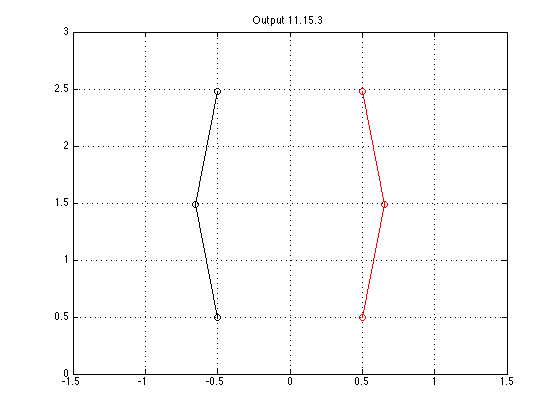``Solution left to the student.``

### Output 11.15.4:

``Solution left to the student.``

### Output 11.15.5:

``Solution left to the student.``

### Output 11.15.6 [Audio]

``Solution left to the student.``

### Output 11.15.7:

``Solution left to the student.``

### Output 11.15.8 [Audio]

``Solution left to the student.``

### Output 11.15.9:

``Solution left to the student.``

### Output 11.15.10:

``Solution left to the student.``

### Output 11.15.11:

``Solution left to the student.``